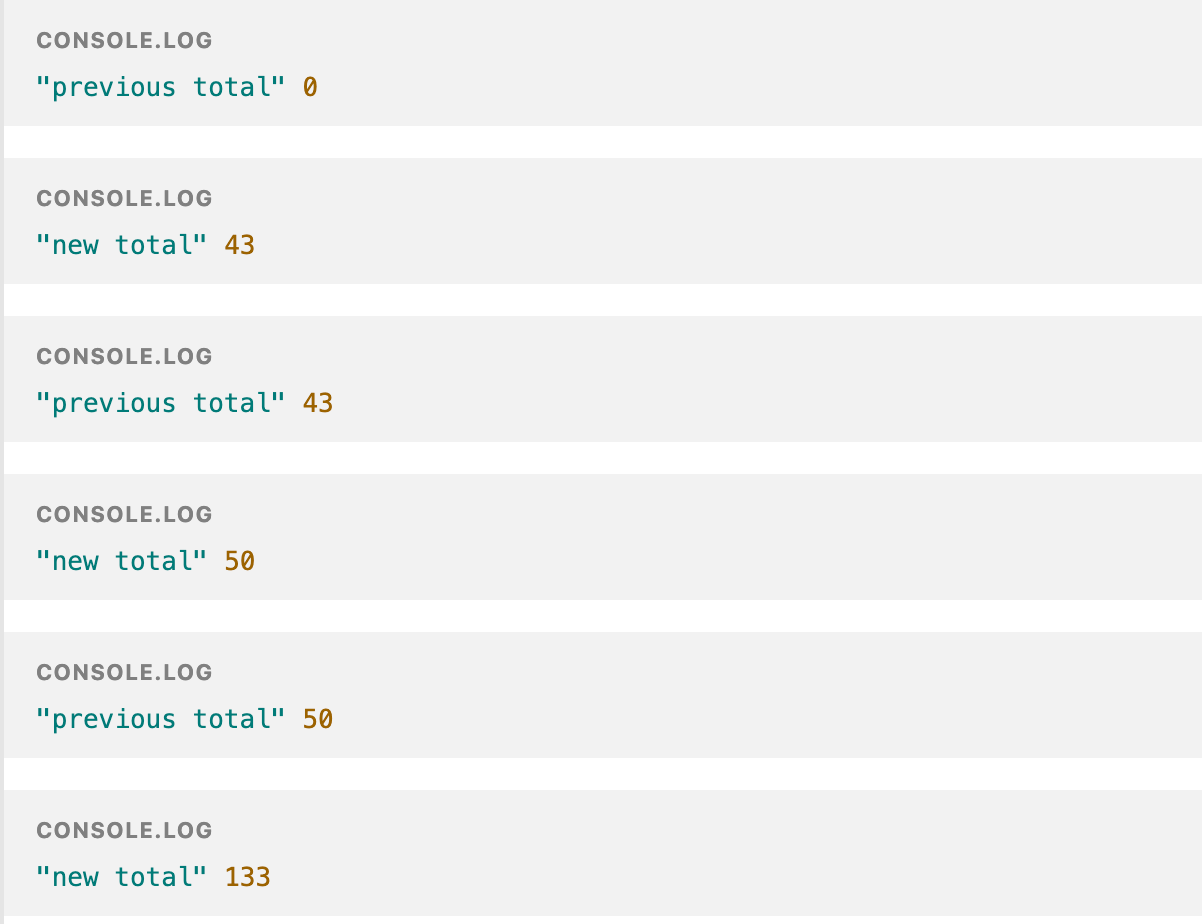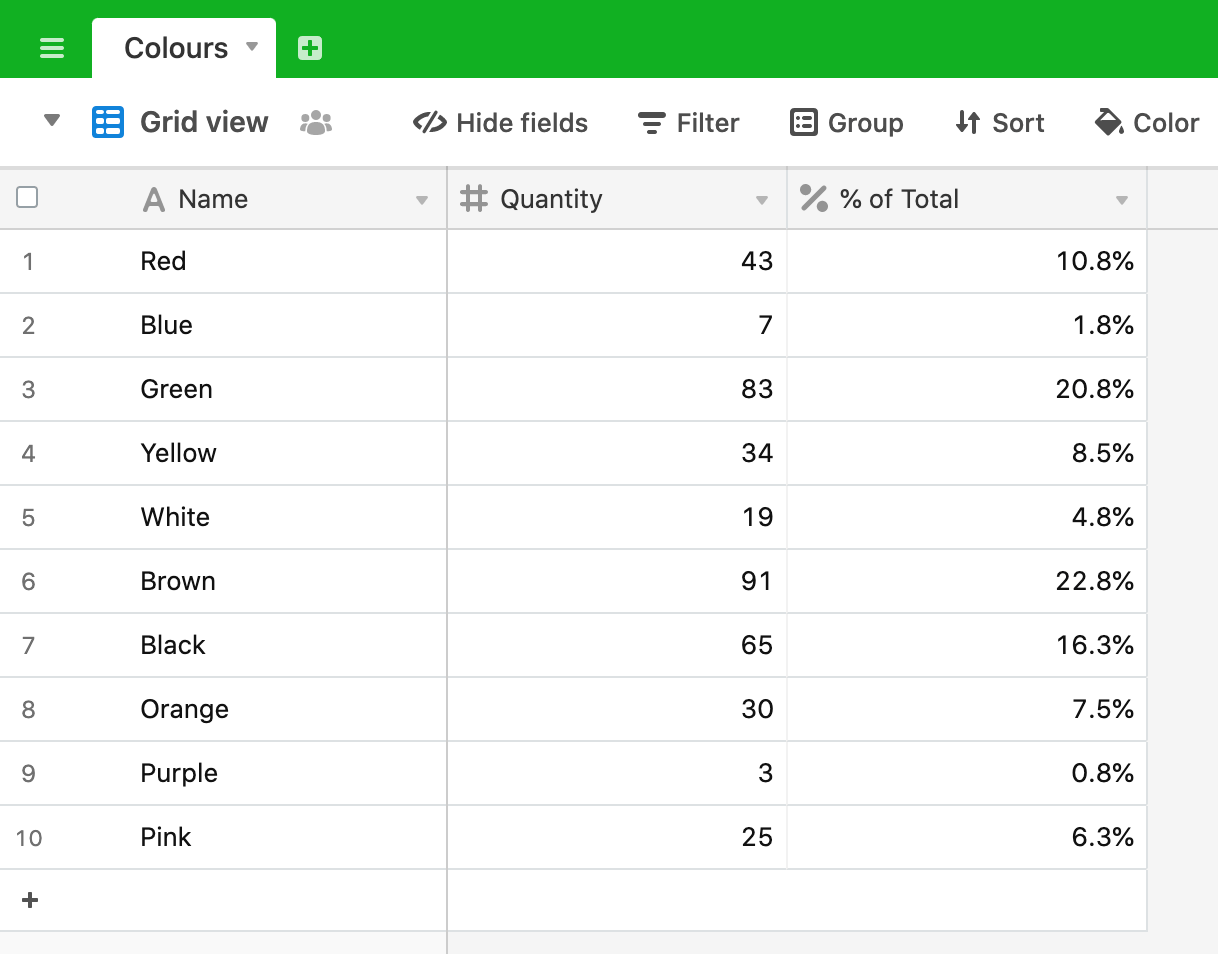# Aggregating numbers

Airtable is great at applying formulas to record values and has ways of grouping and aggregating data, but it doesn’t have a built-in way of comparing each record to a group total. Here’s our problem for this post:Let’s say we have taken a survey to find out the favourite colour of a group of people. Airtable allows us to sum the total number of people - 400 - at the foot of the field, but there isn’t a native way to compare each record to the total to find out the proportion of the total that each colour represents. A perfect job for an Airtable script.

Our method for this script is:

• Calculate the number of favourites recorded (in this case 400)
• Calculate the percentage share of each colour by comparing its score to the total score
• Update the “% of Total” field with the result for each colour

We’ll start this script in a typcial way by defining the table we want to query and querying the records within it:

``````
let table = base.getTable('Colours');
let query = await table.selectRecordsAsync();

``````

We need to sum the records to calculate the total, so let’s define a variable to hold the total for our calculations:

`let total = 0;`

We now need to loop through all of the records and add each “Quantity” value to our total.

``````
for (let record of query.records) {
total = total + record.getCellValue('Quantity');
}

``````

For the coding new-comer, this might look a bit confusing, so let’s break it down.

The `for` loop is simply iterating through all of the records returned by the query. This line:

`total = total + record.getCellValue('Quantity');`

is saying: “the new total equals the previous total plus the “Quantity” value of the current record”. We can make this process clearer by adding some logging to the script before and after the summing task takes place. Modify your `for` loop to this:

``````
for (let record of query.records) {
console.log('previous total', total);
total = total + record.getCellValue('Quantity');
console.log('new total', total);
}

``````

Now run the script and you should get this output:As you can see, the total starts of at `0`. `43` is added giving a new total for iteration 1. On iteration 2 the new total becomes the previous total and we add the second record’s value (`7`) to it to give a new total of `50`. On the 3rd iteration, `50` becomes the previous total and we add `83` to it, giving a new total of `133` and so on. Once the `for` loop has finished we can see that the final total os `400` as we are expecting.

Remove the `previous total` and `new total` logging from the script as we don’t need this to be part of the final version.

Having calcuated the total for all records, we can now loop through the records a 2nd time and calculate the percentage of the total that each record represents. Our 2nd loop looks like this:

``````
for (let record2 of query.records) {
let percentage = record2.getCellValue('Quantity')/total;
await table.updateRecordAsync(record2, {
"% of Total": percentage
})
output.text(`% of Total for \${record2.name} updated!`)
}

``````

Inside the `for` loop, we start by defining a new variable `percentage` and set this to be the “Quantity” value for the current record divided by the total (calculated in the previous loop).

We then update the table for the current record and set the “% of Total” field to be our newly calculated `percentage` value. For good measure, we output some text on each iteration when the record has been updated.

Once the script has finished our table will look like this:and, of course, the total of the “% of Total” field adds up to 100% as you would expect.

So, our final script is:

``````
let table = base.getTable('Colours');
let query = await table.selectRecordsAsync();

let total = 0;

for (let record of query.records) {
total = total + record.getCellValue('Quantity');
}

for (let record2 of query.records) {
let percentage = record2.getCellValue('Quantity')/total;
await table.updateRecordAsync(record2, {
"% of Total": percentage
})
output.text(`% of Total for \${record2.name} updated!`)
}

``````

## Recap

• Looped through the records once to create the total of the “Quantity” field
• Looped through the records a 2nd time to calculate each record’s “Quantity” to “Total” percentage
• Updated the table with the percentage result for each record, notifying the user on each update